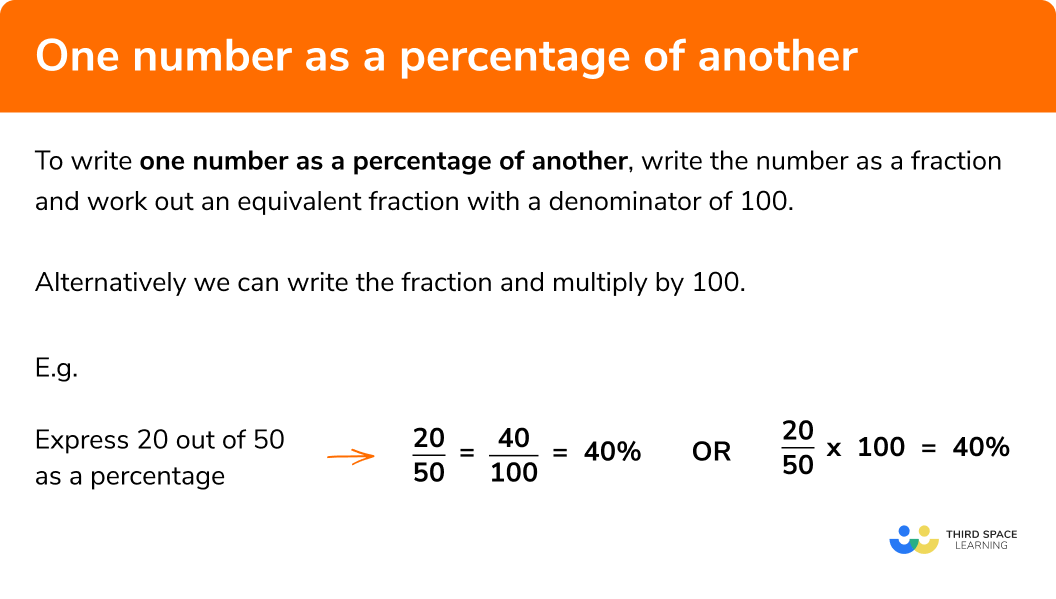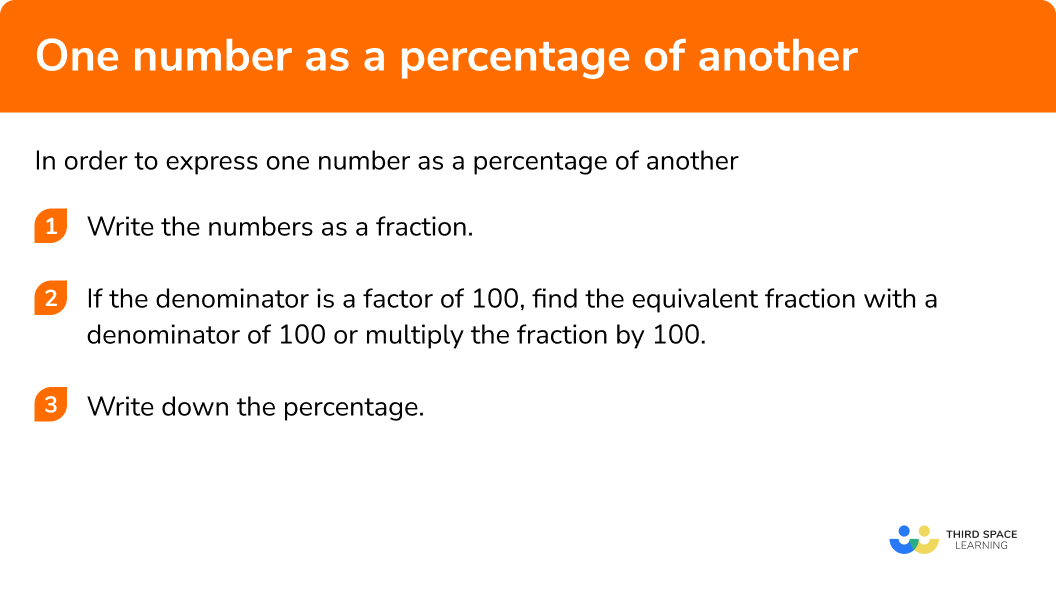GCSE Maths Number FDP Percentages

One Number as a Percentage of Another

# One Number as a Percentage of Another

Here we will learn how to write one number as a percentage of another.
There are also percentages worksheets based on Edexcel, AQA and OCR exam questions, along with further guidance on where to go next if you’re still stuck.

## What is one number as a percentage of another?

One number as a percentage of another is where we have two numbers with one number expressed as a percentage of the other.

To do this we write a fraction and work out an equivalent fraction with a denominator of 100.  This is because percentages are “per one hundred”.

E.g.

Express 20 out of 50 as a percentage

$\frac{20}{50}=\frac{40}{100}=40\%$

Alternatively we can write the fraction and multiply by 100.

E.g.

$\frac{20}{50}\times100=40\%$

So 20 out of 50 is 40%.

### What is one number as a percentage of another?## How to express one number as a percentage of another

Method 1: In order to express one number as a percentage of another

1. Write the numbers as a fraction
2. Find the equivalent fraction with a denominator of 100 (this may take multiple steps)
3. Write down the percentage

### Explain how to express one number as a percentage of another in 3 steps## One number as a percentage of another examples

### Example 1: finding an equivalent fraction with a denominator of 100

Express 23 out of 50 as a percentage.

1. Write the numbers as a fraction

23 out of 50

23 is the numerator and 50 is the denominator.

$\frac{23}{50}$

2Find the equivalent fraction with a denominator of 100

50 is a factor of 100.

$50\times2=100$

$\frac{23}{50}\times\frac{2}{2}=\frac{46}{100}$

3Write down the percentage

$\frac{46}{100}=46\%$

So 23 out of 50 is 46%.

### Example 2: finding an equivalent fraction with a denominator of 100

Express 13 out of 20 as a percentage.

13 out of 20

13 is the numerator and 20 is the denominator.

$\frac{13}{20}$

20 is a factor of 100.

$20\times5=100$

$\frac{13}{20}\times\frac{5}{5}=\frac{65}{100}$

$\frac{65}{100}=65\%$

So 13 out of 20 is 65%.

## How to express one number as a percentage of another

Method 2: In order to express one number as a percentage of another:

1. Write the numbers as a fraction
2. Multiply the fraction by 100
3. Write down the percentage

### Example 3: write the fraction and multiply by 100

Without a calculator – Express 12 out of 30 as a percentage.

12 out of 30

12 is the numerator and 30 is the denominator.

$\frac{12}{30}$

$\frac{12}{30}\times100=\frac{12}{30}\times\frac{100}{1}=40$

When you are not using a calculator, try to cancel common factors in the numerator and denominator.

Here we can cancel with a common factor of 10.

### Example 4: write the fraction and multiply by 100

Without a calculator express 66 out of 150 as a percentage.

66 out of 150

66 is the numerator and 150 is the denominator.

$\frac{66}{150}$

$\frac{66}{150}\times100=\frac{66}{150}\times\frac{100}{1}=44$

When you are not using a calculator, try to cancel common factors in the numerator and denominator.

Here we can cancel with a common factor of 10 and then 3.

### Example 5: write the fraction and multiply by 100

With a calculator express 33 out of 60 as a percentage.

33 out of 60

33 is the numerator and 60 is the denominator.

$\frac{33}{60}$

$\frac{33}{60}\times100=55$

### Example 6: write the fraction and multiply by 100

With a calculator express 39 out of 75 as a percentage.

39 out of 75

39 is the numerator and 75 is the denominator.

$\frac{39}{75}$

$\frac{39}{75}\times100=52$

### Common misconceptions

• Use the same units of measurement for both numbers

Express 300m as a percentage of 2km.

2km = 2000m

$\frac{300}{2000}=\frac{30}{200}=\frac{15}{100}=15\%$

• The first number is usually the numerator, but not always

Of 40, what percent is 24?

The “of” number is always the denominator.

$\frac{24}{40}=\frac{6}{10}=\frac{60}{100}=60\%$

• A percentage does not have to be a whole number, it can also be a decimal number

Express 62 out of 80 as a percentage.

$\frac{62}{80}\times100=77.5$

• Make sure that you have the correct denominator

In a house there are 2 dogs and 3 cats.  What percentage of the pets are cats?

The denominator needs to be the total number of pets. There are 5 pets altogether.

$\frac{3}{5}=\frac{60}{100}=60\%$

• The fraction can be an improper fraction, with the denominator being a smaller number than the numerator

Work out the percentage increase from 40 to 90.

40 is the original amount. This is the denominator.

The increase is 90 – 40 = 50.  This is the numerator.

$\frac{50}{40}\times100=125$

The percentage increase is 125%.

One number as a percentage of another is part of our series of lessons to support revision on percentages. You may find it helpful to start with the main percentages lesson for a summary of what to expect, or use the step by step guides below for further detail on individual topics. Other lessons in this series include:

### Practice one number as a percentage of another questions

1. Without a calculator express 4 out of 10 as a percentage.

4\%40\%6\%60\%Express the quantities as a fraction and multiply by 100 ,

\frac{4}{10} \times 100 = 40\%

2. Without a calculator express 7 out of   20 as a percentage.

7\%35\%28\%27\%Express the quantities as a fraction and multiply by 100 ,

\frac{7}{20} \times 100 = 35\%

3. Without a calculator express 12 out of   50 as a percentage.

62\%38\%12\%24\%Express the quantities as a fraction and multiply by 100 ,

\frac{12}{50} \times 100 = 24\%

4. Express 42 out of 60 as a percentage.

42\%60\%18\%70\%Express the quantities as a fraction and multiply by 100 ,

\frac{42}{60} \times 100 = 70\%

5. Express 36 out of 80 as a percentage.

42\%45\%36\%44\%Express the quantities as a fraction and multiply by 100 ,

\frac{36}{80} \times 100 = 45\%

6. Express 216 out of 300 as a percentage.

84\%36\%72\%18\%Express the quantities as a fraction and multiply by 100 ,

\frac{216}{300} \times 100 = 72\%

### One number as a percentage of another GCSE questions

1.  Gary found 60 trees altogether in a wood.

18 of them were beech trees.

What percentage of the trees were beech trees?

(2 marks)

\frac{18}{60}

(1)

\frac{18}{60}=\frac{3}{10}=\frac{30}{100}=30\%

(1)

2.  With a calculator.

Jack buys a pack of 12 cans of drinks.

The pack costs \pounds 7.32

Jack sells all 12 cans for 70p .

Work out Jack’s percentage profit.

(3 Marks)

12\times70=840

(1)

840-732=108

\frac{108}{732}

(1)

\frac{108}{732}\times100=14.75…=14.8\%

(1)

3. Without a calculator.

Work out the percentage increase from 40 to 240 .

(3 Marks)

240-40=200

(1)

\frac{200}{40}

(1)

\frac{200}{40}\times\frac{100}{1}=500

The percentage increase is 500\%

(1)

### Percentage calculator tips

To calculate with percentages using a calculator you can either use the fraction button or use the divide button.

E.g.
What is 39 out of 75 as a percentage?

Using the fraction button:

$\frac{39}{75}\times100=52$

Using the divide button:

$39\div75\times100=52$

## Learning checklist:

You have now learned how to:

• Express one number as a percentage of another
• Use equivalent fractions to work out one whole number as a percentage of another whole number
• Use a calculator to what percentage one number is of another number accurately

## Still stuck?

Prepare your KS4 students for maths GCSEs success with Third Space Learning. Weekly online one to one GCSE maths revision lessons delivered by expert maths tutors.

Find out more about our GCSE maths tuition programme.